How Cheenta works to ensure student success?
Explore the Back-Story

# TIFR 2014 Problem 22 Solution -An application of Intermediate Value TheoremTIFR 2015 Problem 7 Solution is a part of TIFR entrance preparation series. The Tata Institute of Fundamental Research is India's premier institution for advanced research in Mathematics. The Institute runs a graduate programme leading to the award of Ph.D., Integrated M.Sc.-Ph.D. as well as M.Sc. degree in certain subjects.
The image is a front cover of a book named Introduction to Real Analysis by R.G. Bartle, D.R. Sherbert. This book is very useful for the preparation of TIFR Entrance.

Also Visit: College Mathematics Program of Cheenta

## Problem:

Let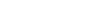be a continuous map such that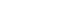for only finitely many values of (x). Which of the following is true?

A. eitherfor all (x) orfor all (x).

B. the map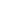is onto

C. the mapis one-to-one

D. none of the above

## Discussion:

Letbe the map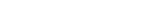. Thenis zero at only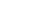.is continuous because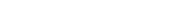is continuous from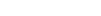. i.e, each arrow is continuous. The first arrow is the projection map, and such maps are always continuous, and the second arrow is just squaring, which is continuous. And composition of continuous functions are continuous, so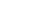is continuous function from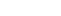. Where here and henceforth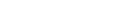.

Similar reasoning will show that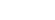is continuous function from.

Sum of continuous functions is continuous, so the map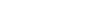is continuous function from.

This functionis not one-one since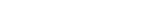and it is not onto since it only takes values in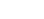.

So we now are sure that B,C are false options.

We will prove A now.

Let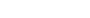and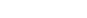be two points in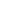such thatand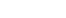.

We will prove that this will imply infinitely many zeros in between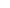and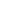. But wait a second... what does between mean in this context? For that we consider the paths betweenand. Note that there are infinitely many paths between any two points in. Further, we can in fact have infinitely many paths completely disjoint except for the initial and final points. We show that corresponding to each path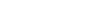which connectsandwe have a zero in the path. Since there are infinitely many disjoint paths, we get infinitely many distinct zeros for.

Now,is a continuous function ,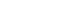,.

Consider the composition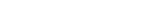.is continuous.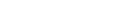and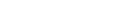.

Therefore by the intermediate value theorem,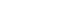for some.

That means,. And using the discussion above we get a contradiction.

This proved the option A.

## Chatuspathi

• What is this topic: Real Analysis
• What are some of the associated concept: Continuity,Intermediate Value Theorem
• Book Suggestions: Introduction to Real Analysis by R.G. Bartle, D.R. SherbertTIFR 2015 Problem 7 Solution is a part of TIFR entrance preparation series. The Tata Institute of Fundamental Research is India's premier institution for advanced research in Mathematics. The Institute runs a graduate programme leading to the award of Ph.D., Integrated M.Sc.-Ph.D. as well as M.Sc. degree in certain subjects.
The image is a front cover of a book named Introduction to Real Analysis by R.G. Bartle, D.R. Sherbert. This book is very useful for the preparation of TIFR Entrance.

Also Visit: College Mathematics Program of Cheenta

## Problem:

Letbe a continuous map such thatfor only finitely many values of (x). Which of the following is true?

A. eitherfor all (x) orfor all (x).

B. the mapis onto

C. the mapis one-to-one

D. none of the above

## Discussion:

Letbe the map. Thenis zero at only.is continuous becauseis continuous from. i.e, each arrow is continuous. The first arrow is the projection map, and such maps are always continuous, and the second arrow is just squaring, which is continuous. And composition of continuous functions are continuous, sois continuous function from. Where here and henceforth.

Similar reasoning will show thatis continuous function from.

Sum of continuous functions is continuous, so the mapis continuous function from.

This functionis not one-one sinceand it is not onto since it only takes values in.

So we now are sure that B,C are false options.

We will prove A now.

Letandbe two points insuch thatand.

We will prove that this will imply infinitely many zeros in betweenand. But wait a second... what does between mean in this context? For that we consider the paths betweenand. Note that there are infinitely many paths between any two points in. Further, we can in fact have infinitely many paths completely disjoint except for the initial and final points. We show that corresponding to each pathwhich connectsandwe have a zero in the path. Since there are infinitely many disjoint paths, we get infinitely many distinct zeros for.

Now,is a continuous function ,,.

Consider the composition.is continuous.and.

Therefore by the intermediate value theorem,for some.

That means,. And using the discussion above we get a contradiction.

This proved the option A.

## Chatuspathi

• What is this topic: Real Analysis
• What are some of the associated concept: Continuity,Intermediate Value Theorem
• Book Suggestions: Introduction to Real Analysis by R.G. Bartle, D.R. Sherbert

This site uses Akismet to reduce spam. Learn how your comment data is processed.

### Knowledge Partner